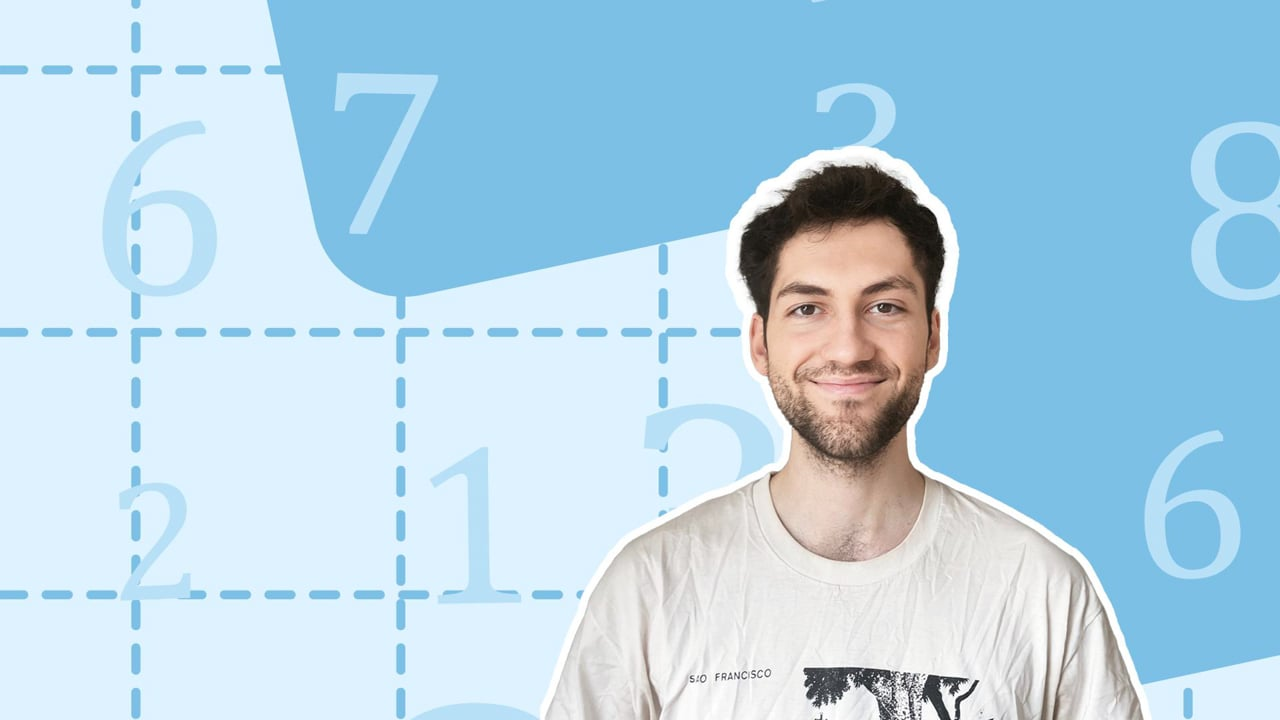Chapter overviewMaths

Number and place value

Multiplication and division

Fractions

Measurement

Geometry - properties of shapes

Statistics

Maths0%

Summary

## ​​In a nutshell

Since numbers are expressed using ones, tens, hundreds, etc, when adding numbers, these categories can be ordered into columns, making the addition easier to follow and process.

## The columns

Columns go up and down. For column addition, if you place one number above another, lining them up from the right, the digits will align. These can be thought of as columns.

##### Example 1

To calculate

$15712+2163$​​

using the column addition method, you would set them up as follows:

Notice that the units of each number lines up in a column. The tens in each number line up in another column, and so on. This is why it is important to line the numbers up from the right and not from the left.

Now that the corresponding digits are aligned in columns, they can be added. You start from the right with the ones. The sum of the ones goes beneath, in the same column. Then move to the left on to the tens. Continue this process until you have reached the very left.

##### Example 2

Calculate

$15712+2163$​​

As seen above, this can be done using the column method:

## Carrying forward

There's an important reason for working from right to left. If your column adds to a number bigger than $9$, you can no longer fit the value beneath. Hence you "carry forward" what won't fit.

##### Example 3

Calculate

$358+294$​​

When you add the ones ($8$ and $4$), you get a two-digit number. But the column method relies on working with single digits. Hence, carry the greater digit (in this case the $1$ that represents the ten) to the left and leave the lower digit (the $2$ that represents the ones). In the diagram above, the carried digit is written above the column to the right (the tens column). This is to indicate that when you add the tens, you also add this digit.

Hence when you move to adding the tens, you not only add $5$ and $9$, but also the carried $1$ from the previous step. The same thing happens with the tens column, so a $1$ is carried to the left to the hundreds column.

So

$358+294=\underline{652}$

FAQs

• Question: In column addition, why do you work from right to left?

Answer: There's an important reason for working from right to left. If your column adds to a number bigger than 9, you can no longer fit the value beneath. Hence you "carry forward" what won't fit.

• Question: How do you do column addition?

Answer: You start from the right with the units. The sum of those units goes beneath, in the same column. Then move to the left on to the tens. Continue this process until you have reached the very left.

• Question: What is a column in column addition?

Answer: Columns go up and down. For column addition, if you place one number above another, lining them up from the right, the digits will align. These can be thought of as columns.

Theory

Exercises